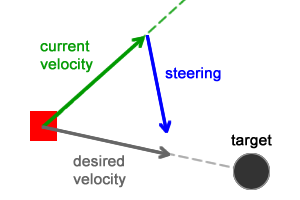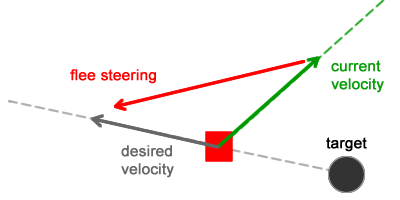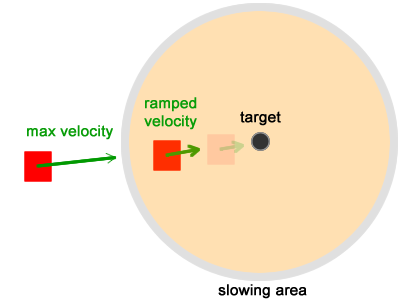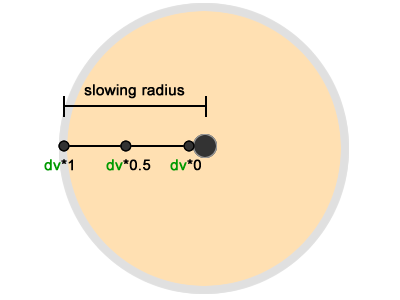# Steering Behaviors: Flee and Arrival 逃逸和到达

Flee

```desired_velocity = normalize(target - position) * max_velocity
steering = desired_velocity - velocity```

desired_velocity在这里就是离目标位置的最短路径，根据目标点的位置和个体的位置相减得出，代表了一个以个体位置为起点，朝向目标点的力。Flee行为也用到这两个力，区别是它们被调整为使游戏个体远离目标位置。Flee行为中 desired_velocity 根据个体的位置和目标点的位置相减得出，产生了一个以目标点为起点，朝向个体的力。

```desired_velocity = normalize(position - target) * max_velocity
steering = desired_velocity - velocity```

`flee_desired_velocity = -seek_desired_velocity`

Arrival行为会规避个体移动超过目标点的现象，当个体靠近目标点时，它会使个体减速，最终停在目标点上。```/ If (velocity + steering) equals zero, then there is no movement
velocity = truncate(velocity + steering, max_speed)
position = position + velocity

function truncate(vector:Vector3D, max:Number) :void {
var i :Number;
i = max / vector.length;
i = i < 1.0 ? i : 1.0;
vector.scaleBy(i);
}```

```// Calculate the desired velocity
desired_velocity = target - position
distance = length(desired_velocity)

// Check the distance to detect whether the character
// is inside the slowing area
// Inside the slowing area
desired_velocity = normalize(desired_velocity) * max_velocity * (distance / slowingRadius)
} else {
// Outside the slowing area.
desired_velocity = normalize(desired_velocity) * max_velocity
}

// Set the steering based on this
steering = desired_velocity - velocity```

steering = desired_velocity – velocity 如果距离比slowingRadius大，这意味着个体离目标点还远，那它的速度将保持为max_velocity。```steering = desired_velocity - velocity
velocity = truncate (velocity + steering , max_speed)
position = position + velocity```